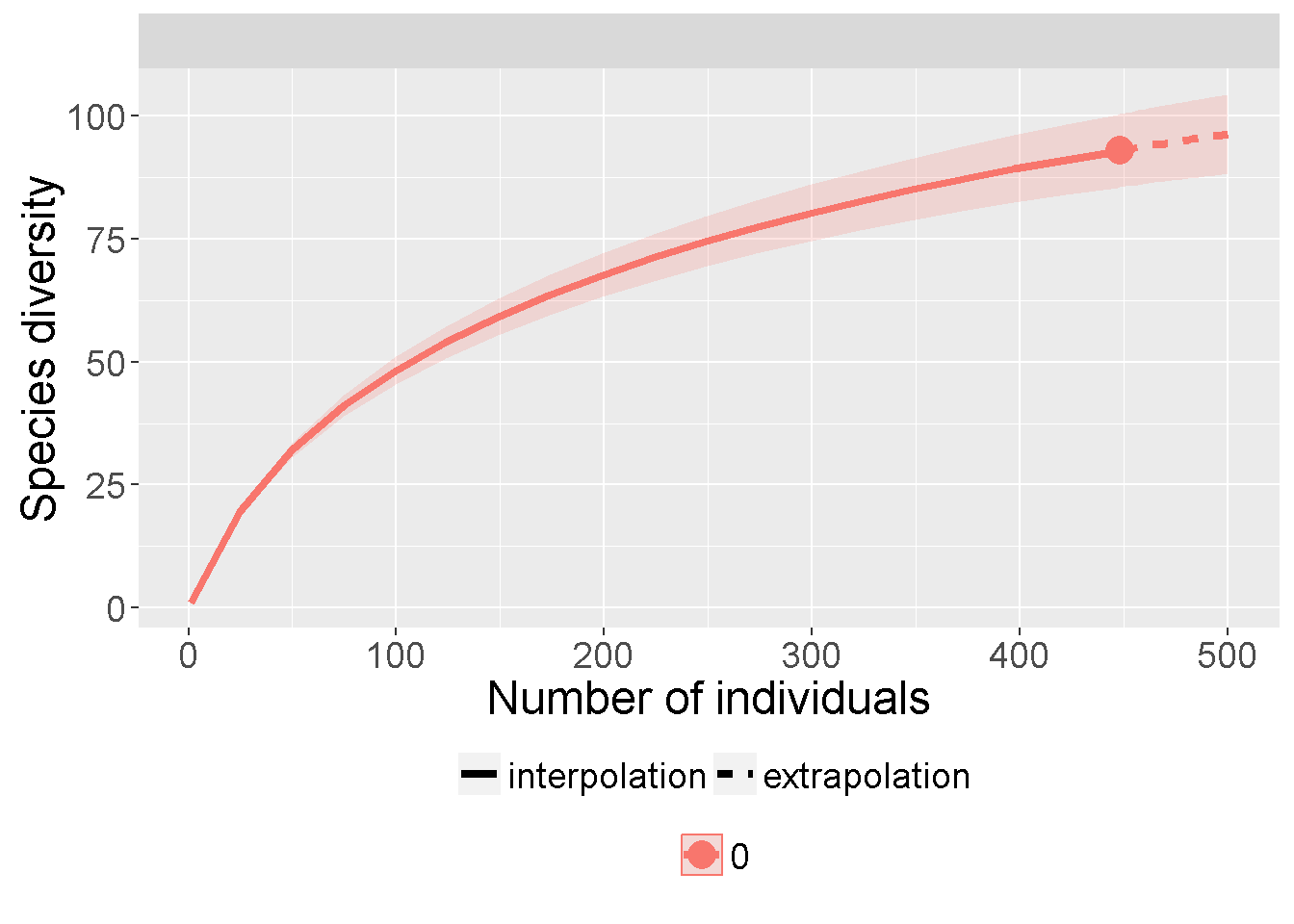Commands retated to species accumulation curves & rarefaction

• specaccum(): “Function specaccum finds species accumulation curves or the number of species for a certain number of sampled sites or individuals.”"
• rarefy(): “Rarefied species richness for community ecologists.”
• specnumber(): number of species per row inf site x spp matrix
• rarecurve()
• estimateR(): is based on abundances (counts) on single sample site.
• diversity(): diversity by site

Species accumulation curve: species richness

library(vegan)
data(BCI)

#Vegan data is a SITE x SPECIES matrix
## 1                  0                     0                     0
## 2                  0                     0                     0
## 3                  0                     0                     0
## 4                  0                     0                     0
## 5                  0                     0                     0
## 6                  0                     0                     0
#Default specaccum
sp1 <- specaccum(BCI)

# specaccum w/ method = "collector"
sp2 <- specaccum(BCI, method = "collector")

par(mfrow = c(2,2), mar = c(4,2,2,1))
plot(sp1, main = "Default: plot( specaccum(BCI) )")
plot(sp2, main = "method = collector")
plot(sp1, ci.type="poly", col="blue", lwd=2, ci.lty=0, ci.col="lightblue",
main = "Default: Prettier CI")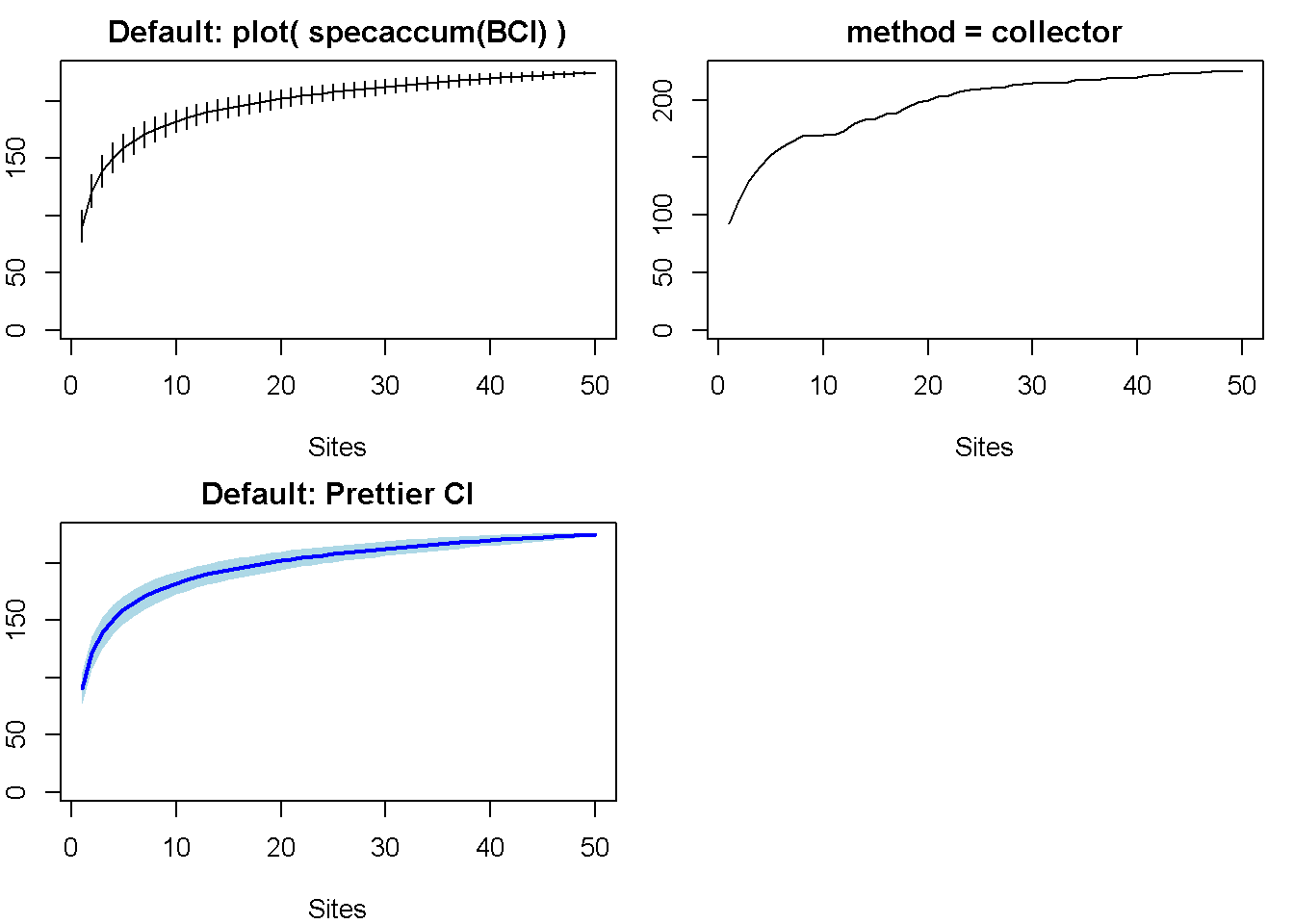Rarefaction curves with vegan: comparisons between surveys/studies (?)

library(vegan)
data(BCI)
#total number of species at each site (row of data)
S <- specnumber(BCI)

# Number of INDIVIDULS per site (?)
raremax <- min(rowSums(BCI)) # = 340;

# rarefy, w/ raremax as input (?)
Srare <- rarefy(BCI, raremax)

#Plot rarefaction results
par(mfrow = c(1,2))
plot(S, Srare, xlab = "Observed No. of Species",
ylab = "Rarefied No. of Species",
main = " plot(rarefy(BCI, raremax))")
abline(0, 1)
rarecurve(BCI, step = 20,
sample = raremax,
col = "blue",
cex = 0.6,
main = "rarecurve()")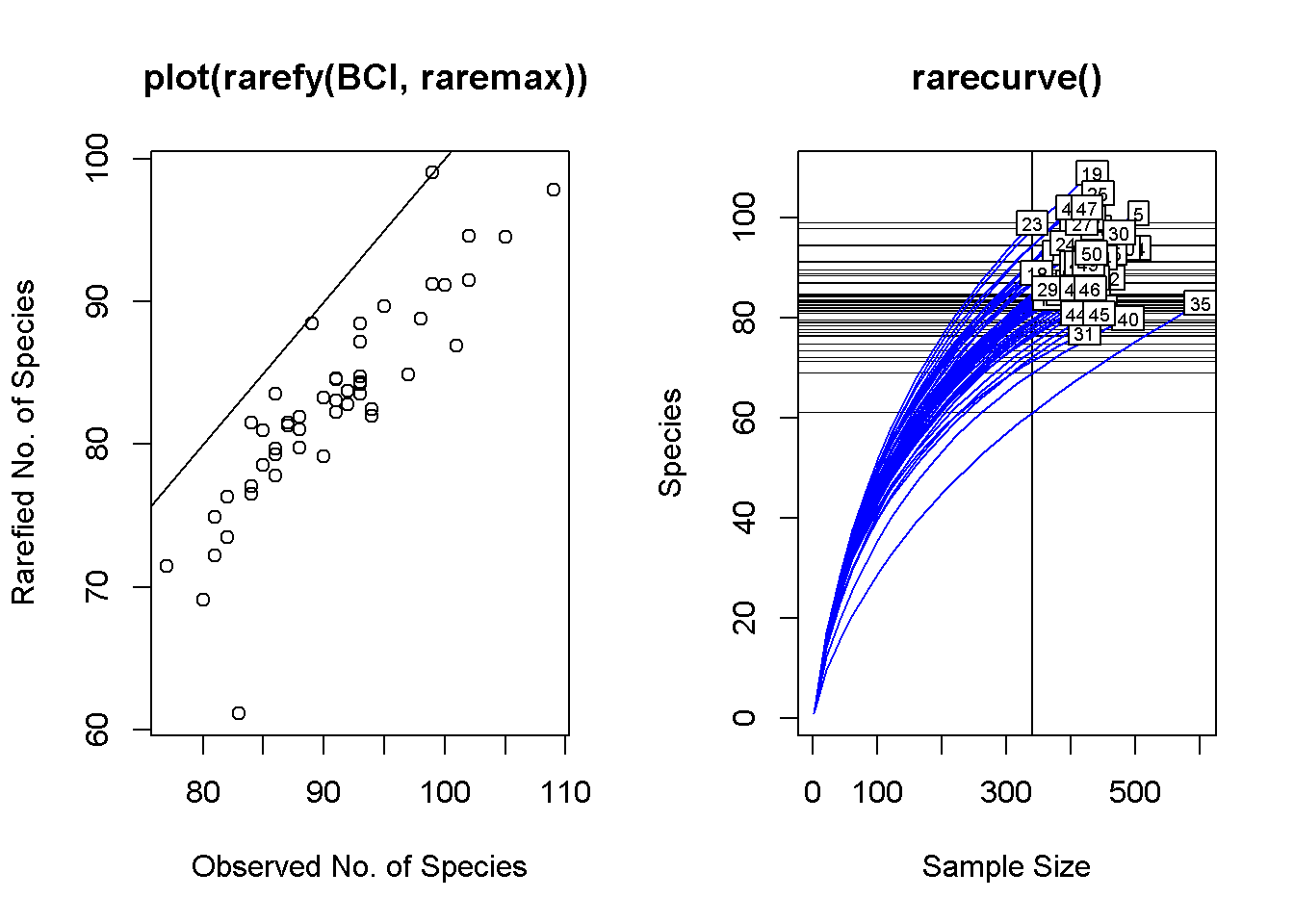rarecurve(BCI[1:5,], step = 20, sample = raremax, col = "blue", cex =      0.6,
main = "rarecurve() on subset of data")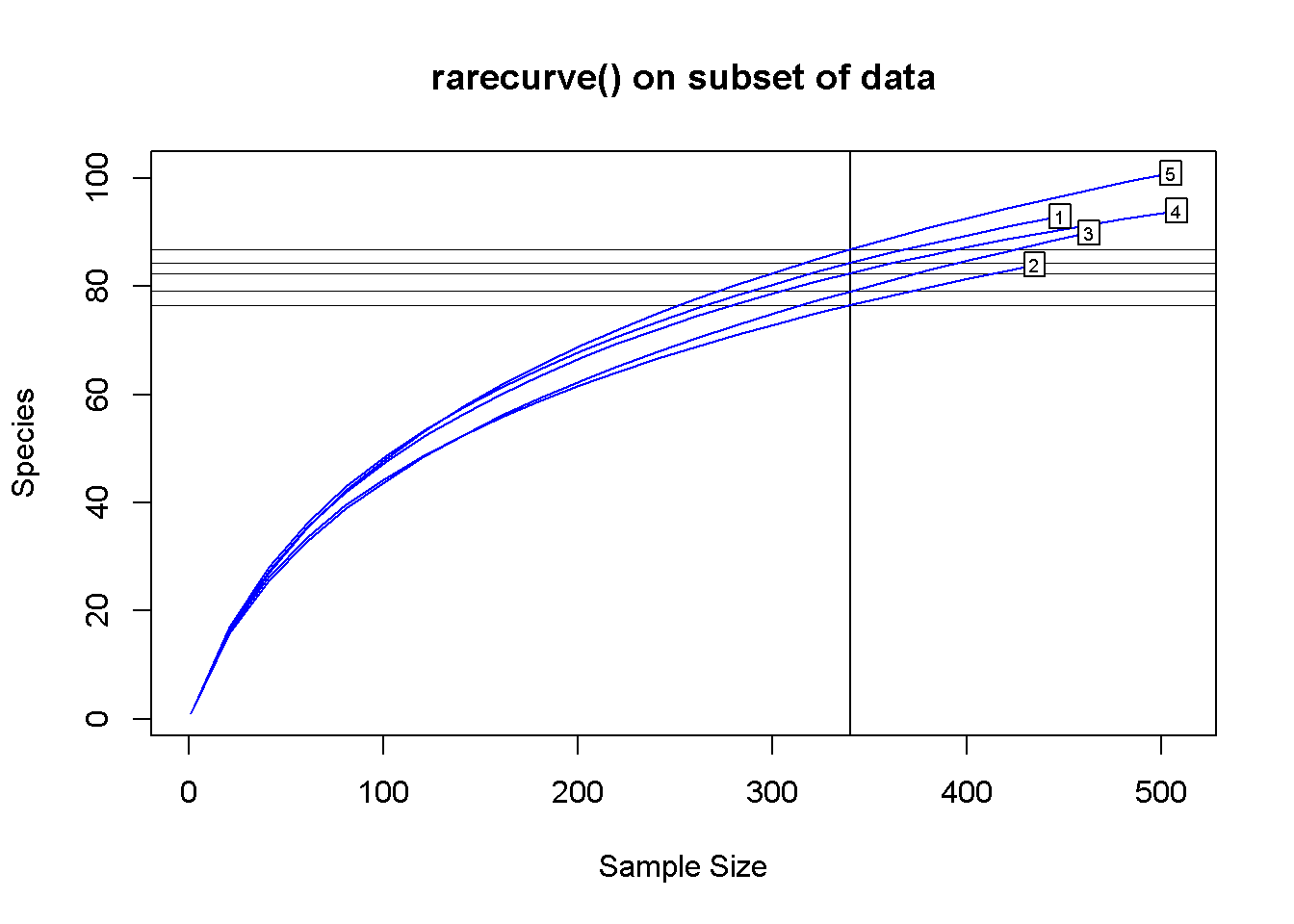Different setting for vegan::specaccum

specaccum() has several different methods * default = “exact” ; finds the expected (mean) species richness * collector: “adds sites in the order they happen to be in the data” * rarefaction: “finds the mean when accumulating individuals instead of sites”

Plotting an object produced by specaccum() can take three “xvar=” arguements * xvar = c(“sites”) * …individuals * …effort

par(mfrow = c(2,3), mar = c(2,1,2,1))
#Default
plot(specaccum(BCI), main = "All Defaults ")
## Warning in cor(x > 0): the standard deviation is zero
# method = "collector"
plot(specaccum(BCI, method = "collector"),main = "method = collector")

# method = "rarefaction"
plot(specaccum(BCI, method = "rarefaction"),main = "method = rarefaction")
legend("bottomleft", legend = "Note x-axis is # of plots")

# method = "rarefaction"
plot(specaccum(BCI,
method = "rarefaction"),
xvar = "individuals",
main = "rarefaction, xvar = individuals")
legend("bottomleft", legend = "Note x-axis has large range")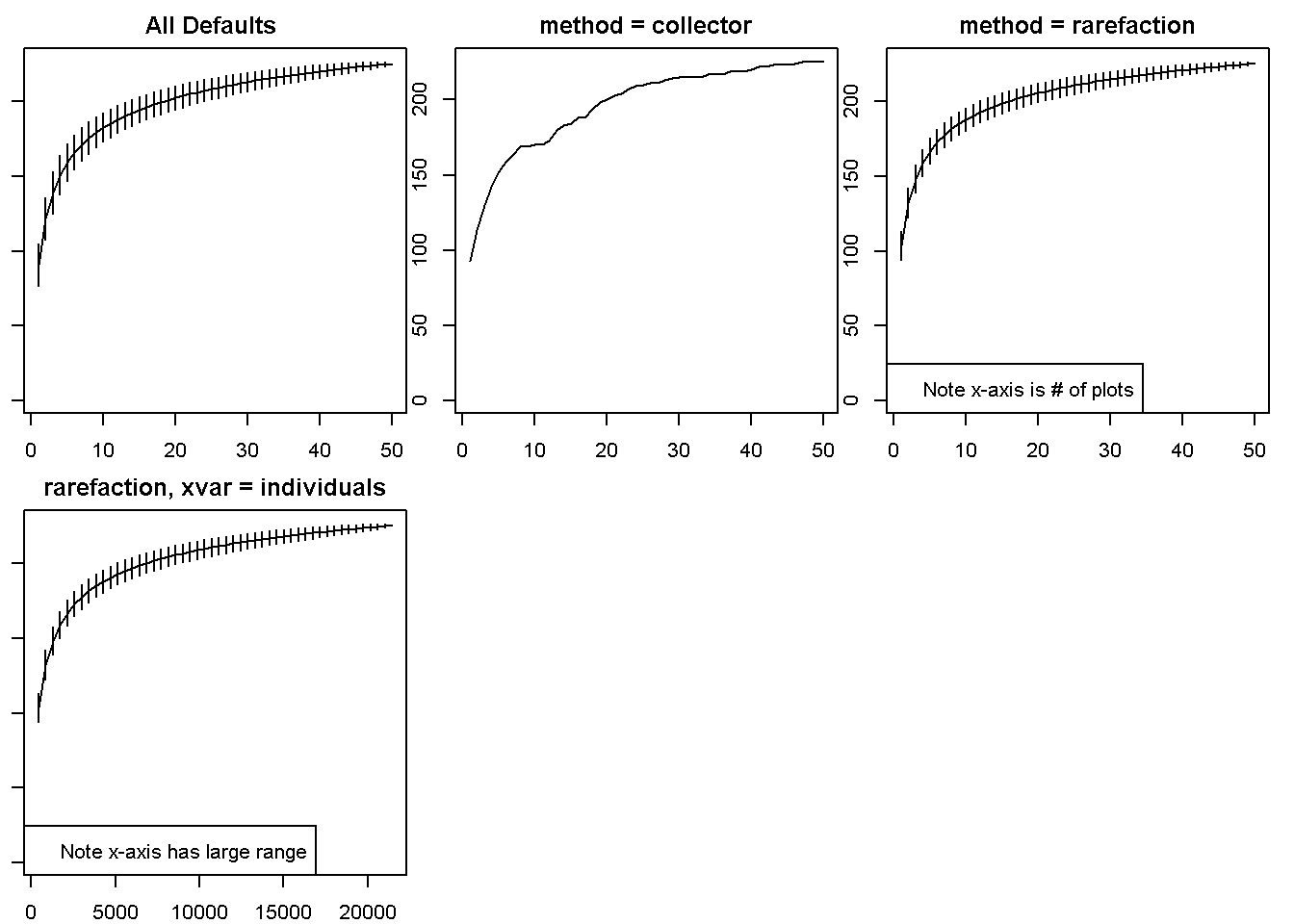Rarefaction commands in vegan

• rarefy
• rrarefy(): generates one randomly rarefied community data frame or vector
• drarefy(): returns probabilities that species occur in a rarefied community of size
• rarecurve(): draws a rarefaction curve for each row of the input data
• rareslope(): calculates the slope of rarecurve

iNEXT by Chao et al

library(iNEXT)
library(ggplot2)

Hill numbers

• 1 = species richness
• 2 = Shannon div, exp(Shannon entropy)
• 2 = Simpson div, 1/(Simpson concentratino)

Data types in iNEXT

• Individual-based abundance data (datatype=“abundance”) “Input data for each assemblage/site include samples species abundances in an empirical sample of n individuals (”reference sample“). When there are N assemblages, input data consist of an S by N abundance matrix, or N lists of species abundances.”

• Sampling-unit-based incidence dat“:
• Incidence-raw data (datatype=“incidence_raw”): “for each assemblage, input data for a reference sample consist of a species-by-sampling-unit matrix; when there are N assemblages, input data consist of N lists of matrices, and each matrix is a species-by-sampling-unit matrix.”

• Incidence-frequency data (datatype=“incidence_freq”): “input data for each assemblage consist of species sample incidence frequencies (row sums of each incidence matrix). When there are N assemblages, input data consist of an S by N matrix, or N lists of species incidence frequencies. The first entry of each list must be the total number of sampling units, followed by the species incidence frequencies.”

# The spider data is in a list
data(spider)
str(spider)
## List of 2
##  $Girdled: num [1:26] 46 22 17 15 15 9 8 6 6 4 ... ##$ Logged : num [1:37] 88 22 16 15 13 10 8 8 7 7 ...
# each component of the list is just a vector of abundances
spider$Girdled ##  46 22 17 15 15 9 8 6 6 4 2 2 2 2 1 1 1 1 1 1 1 1 1 ##  1 1 1 # call iNEXT on the list spider.all <- iNEXT(spider, q=0, datatype="abundance") # call iNEXT a vector spider.girdled <- iNEXT(spider$Girdled, q=0, datatype="abundance")

Try it on the BCI data. Need to remova any zeros and coerce into a vector.

library(vegan)
BCI.test.no.zero <- unlist(BCI[1,])

i.zero <- which(BCI.test.no.zero == 0)
BCI.test.no.zero <- BCI.test.no.zero[-i.zero]

iNEXT(as.vector(BCI.test.no.zero), q=0, datatype="abundance")
## Compare 1 assemblages with Hill number order q = 0.
## $class: iNEXT ## ##$DataInfo: basic data information
##     site   n S.obs    SC f1 f2 f3 f4 f5 f6 f7 f8 f9 f10
## 1 site.1 448    93 0.931 31 18  9  6  6  4  1  1  1   1
##
## $iNextEst: diversity estimates with rarefied and extrapolated samples. ## m method order qD qD.LCL qD.UCL SC SC.LCL SC.UCL ## 1 1 interpolated 0 1.000 1.000 1.000 0.023 0.020 0.027 ## 10 224 interpolated 0 71.263 65.623 76.904 0.863 0.843 0.883 ## 20 448 observed 0 93.000 84.663 101.337 0.931 0.910 0.952 ## 30 660 extrapolated 0 104.269 93.224 115.313 0.960 0.938 0.982 ## 40 896 extrapolated 0 111.305 96.847 125.763 0.978 0.959 0.998 ## ##$AsyEst: asymptotic diversity estimates along with related statistics.
##                   Observed Estimator Est_s.e. 95% Lower 95% Upper
## Species Richness    93.000   119.635   12.553   104.072   157.075
## Shannon diversity   55.613    64.503    3.376    57.886    71.120
## Simpson diversity   39.416    43.121    2.688    39.416    48.391
##
## NOTE: Only show five estimates, call iNEXT.objext\$iNextEst. to show complete output.

iNEXT graphics

With the spider data

out <- iNEXT(spider, q=0, datatype="abundance")

# Sample-size-based R/E curves, separating by "site""
ggiNEXT(out, type=1, facet.var="site")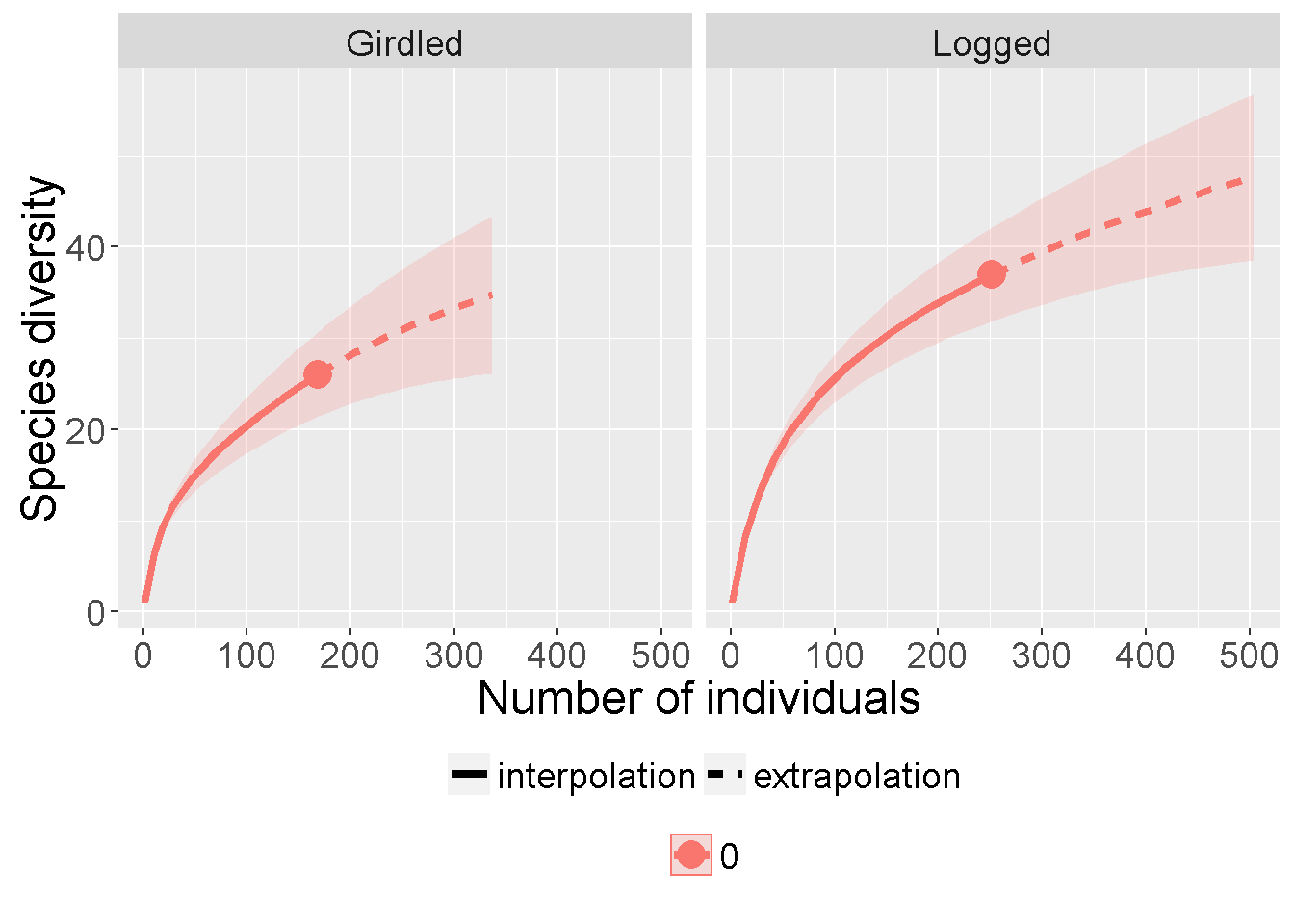ggiNEXT(out, type=1)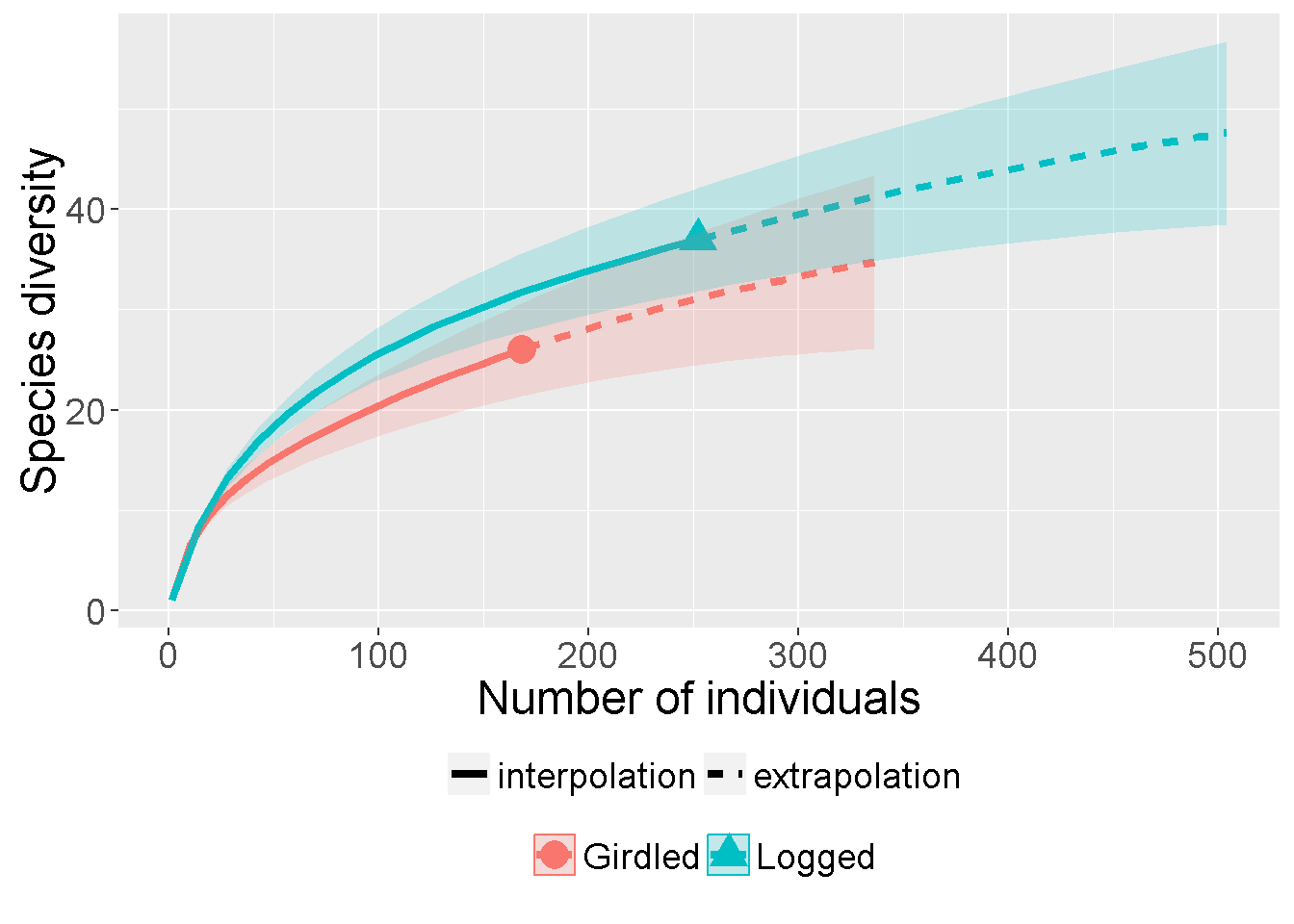With BCI

out <- iNEXT(BCI.test.no.zero, q=c(0), datatype="abundance", endpoint=500)

ggiNEXT(out, type=1, facet.var="site")